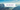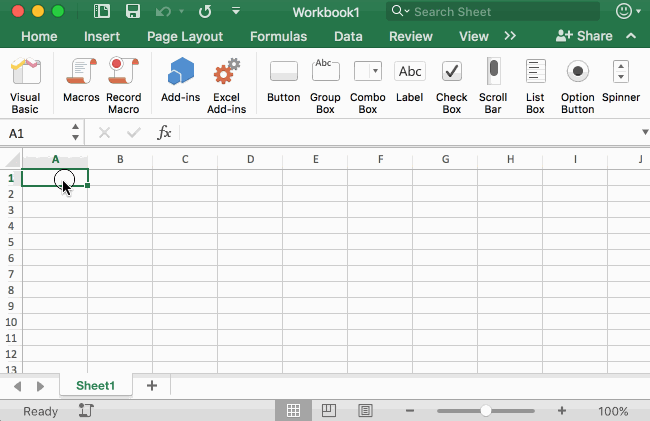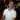# Intro to the VBA Range Object

July 19, 2017

The VBA Range object is the most heavily-used VBA object type in any macro out there. After you record some macros, it will become extremely obvious how often you will use the Range object in Excel VBA. The main reason is that the Range object represents the cells in your worksheet, which we all know is the livelihood of Excel workbooks. There are so many things to talk about with Excel Ranges and originally I had a very, very long post about this topic. However, I decided to try to keep this as simple as possible and I’ll break up this post into several other posts to make them more consumable. I hope you enjoy this series of articles.

Also, if you’re not too familiar with VBA, please see my VBA for Beginners post.

## What is a Range?

Think of a Range like a single cell or set of cells. The word “Range” might even be short for “Cell Range” because it represents a collection (i.e. range) of cells.

A range can be a single cell, multiple cells on the same worksheet, or even a set of 3D cells.

### A Simple Example

If you’ve ever recorded a macro before, then you have inevitably run across the Range object in VBA code.

Here’s a quick example. I recorded a macro that did the following:Here’s a breakdown of what I did:

• Hit `Record Macro`
• Pressed `OK`
• Select cell `B1`
• Type `"Hi"` and press enter
• Select cell `B1` again
• Used the Fill Handle to copy cell `B1`’s value down to cell `B11`
• Pressed Stop Recording

Here’s the code that macro created for us:

``````Sub Macro1()
'
' Macro1 Macro
'

'
Range("B1").Select
ActiveCell.FormulaR1C1 = "Hi"
Range("B1").Select
Selection.AutoFill Destination:=Range("B1:B11"), Type:=xlFillDefault
Range("B1:B11").Select
End Sub``````

As you can see in the code, it follows the steps that I took in the Macro Recorder after I pressed OK to start recording:

Action VBA Result
Select cell `B1` `Range("B1").Select`
Type `"Hi"` and pressed Enter `ActiveCell.FormulaR1C1 = "Hi"`
Select cell `B1` again `Range("B1").Select`
Used the Fill Handle to copy cell `B1`’s value down to cell `B11`  `Selection.AutoFill Destination:=Range("B1:B11"), Type:=xlFillDefault`

Let’s focus on the`Range("B1").Select` part of the code. How does this work?

The part of the code `Range("B1")` is a built-in function that tells Excel “please give me this Range.” After that, we can do different things with that range such as Select it (as shown in this example), add a formula to it, format it, etc.## Using the VBA Range Function

The Range function has a couple of different ways to use it that we’ll cover quickly.

### Range(arg)

The first method to using Ranges that we’ll discuss is the following:

``Range(_arg_)``

Where `_arg_` is the name of the range. This is a string representation that can be any of the following:

• A range reference, like `A1`, `A1:B10`, or multiple cell collections like `A1:B10, C1:D10`
• A named range, like `MyNamedRange`
• Note that you cannot use R1C1 notation in the string here (more on this later).

A simple example is from the above, such as `Range("B1")`.

Note that `Range(_arg_)` is actually shorthand for `ActiveSheet.Range(_arg_)`, meaning that anytime you use `Range(_arg_)` you are referring to the active worksheet. You can change this behavior by specifying the worksheet you want to use, such as `Worksheets("MyWorksheet").Range("A1")` so that no matter what sheet is selected, you will always use the `A1` range in MyWorksheet.

### Range(Range, Range)

In this method, you can pass in two other ranges or two objects that represent ranges (like `ActiveCell` and `Selection`):

`Range(_Range, Range_)`

Each Range parameter inside the main Range function represents any cell or set of cells. The easiest way to think of this is that each Range parameter represents a corner of the entire range that you want to represent. When you lay out the two corners, the overall range will be everything between those two corners.

Examples:

Code Meaning
`Range(Range("A1"), Range("B10")).Select` This will select cells `A1` to `A10`. The `Range("A1")` represents one corner and the `Range("B10")` represents the other corner. When you put those two corners together, you get the overall range of `A1:B10`.
`Range(ActiveCell, Range("A1")).Select` This will select from the `ActiveCell` to `A1`. If your `ActiveCell` is `B10`, then your overall selection will be `B10:A1` (again, think of these as two corners that you put together).

Side note: in an Excel formula, you can actually put ranges like this. If you put `=Sum(B10:A1)` Excel will happily accept that, but it will change your formula to `=Sum(A1:B10)`.

As with `Range(arg)`, the `Range(Range, Range)` function is shorthand for `ActiveSheet.Range(Range, Range)`.

## Objects that are the VBA Range Type

There are some objects in VBA that are actually of the Range type.

VBA Range Object Definition
`Selection` A Range object that represents your entire cell selection. If you selected cells `A1:A10`, then `Selection` is a Range that refers to cells `A1:A10`.
`ActiveCell` Unlike Selection, `ActiveCell` is a Range object that represents the first-selected cell on your worksheet. If you select cells `A1:A10`, the `ActiveCell` would be `A1` since that is the first cell you selected. (You can also see the difference because cell `A1` is not dimmed like cells `A2:A10`)

## Where to go from here

Again, I wanted to keep this post short and to the point by giving an intro to the VBA Range object. From here, I recommend playing around with some of your existing macros, or create some new ones. Try to change the macros by doing one of the following:

• Change any `Selection` to a `Range(Range, Range)` function
• Change any `ActiveCell` to a `Range(arg)` function

Experiment and see what works and what doesn’t work. I’ll be back with more details on more VBA Range functions, like the `Worksheet.Cells` function and the `Range.Offset` function.

Wow, you read the whole article! You know, people who make it this far are true learners. And clearly, you value learning. Would you like to learn more about Excel? Please consider supporting me by buying me a coffee (it takes a lot of coffee to write these articles!).Written by Joseph who loves teaching about Excel.